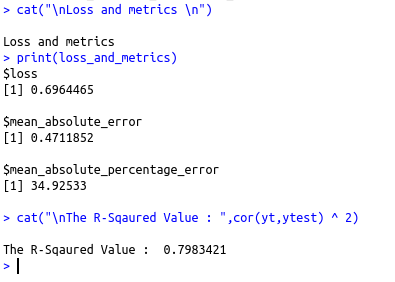• #5, First Floor, 4th Street Dr. Subbarayan Nagar Kodambakkam, Chennai-600 024 Landmark : Samiyar Madam
• pro@slogix.in
• +91- 81240 01111

### How to find the time required for the servo system to respond to a step change in a position set point using deep neural network in R?

###### Description

To find the time required for the servo system to respond to a step change in a position set point using deep neural network in R

#### Functions Used

model layer_dense() – Add a input,output or hidden layer
layer_activation() – Activation function for the layer
compile() – To compile the model
fit() – To fit the model using the train set
predict() – To predict using the test set
evaluate() – To evaluate the loss and metrics

#### Libraries Required :

library(keras)

###### Process

Convert the categorical variables to equivalent numeric classes

Split the data set as train set and test set

Initialize the keras sequential model

Build the model with input layers,hidden layers and output layer as per the data size along with the activation function(here 4 I/P layer with relu activation and 1 O/P layer with no activation(for linear))

Compile the model with required loss,metrics and optimizer(here loss=mean square error,optimizer=adam,metrics=mae,mape)

Fit the model using the train set

Predict using the test set

Evaluate the metrics

###### Sapmle Code

library(caret)
library(keras)
#convert Categorical data to numeric classes
data\$Motor=factor(data\$Motor,labels=c(0:4))
data\$Screw=factor(data\$Screw,labels=c(0:4))
#To Split 80% of data as training data
smp_size train_ind #Train set
train_data<-data[train_ind,]
xtrain=train_data[,1:4]
ytrain=train_data\$Class
#Test set
test=data[-train_ind,]
xtest=test[,1:4]
ytest=test\$Class
#defining a keras sequential model
model %
layer_dense(units = 100, input_shape = 4) %>%
layer_dropout(rate=0.4)%>%
layer_activation(activation = ‘relu’) %>%
layer_dense(units = 1)

#compiling the defined model with metric = accuracy and optimiser as adam.
model %>% compile(
loss = ‘mean_squared_error’,
metrics = c(‘mae’,’mape’)
)
#Summary of the model
summary(model)

#fitting the model on the training dataset
model %>% fit(as.matrix(xtrain), ytrain, epochs = 1000)
#Predict using the Test data
yt=predict(model,as.matrix(xtest))

#Evaluating model on the cross validation dataset
loss_and_metrics % evaluate(as.matrix(xtest), ytest)
cat(“\nLoss and metrics \n”)
print(loss_and_metrics)
cat(“\nThe R-Sqaured Value : “,cor(yt,ytest) ^ 2)

###### Screenshots